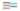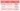# How to do Power Training

‘How to do Power Training?’ Is a question that gets asked very often and there seems to be a lot of confusion about what Power Training is and how to perform Power Training effectively.

In order to understand ‘How to do Power Training’ you need to understand what Power is. I have covered that in previous articles The 101 of Power Training for Beginners and Power Training vs Strength Training – what is the difference between Strength Training and Power Training? if you have read those, feel free to jump to the next paragraph.

### What is Power Training

The physical formula for Power is, Power equals Work divided by Time (P = W / t) or Power equals Force times velocity (P = F * v).

How is Work divided by time the same as Force multiplied by velocity?

Check out the image below.Power equals Work divided by time (P = W / t), where Work equals Force times distance (W = F * d). Consequently, the formula can be displayed as Power equals Force times distance divided by time (P = F * d / t). Distance divided by time is velocity (v = d / t), therefore you can replace distance divided by time with velocity and get to the formula Power equals Force multiplied by velocity (F = F * v)

From the formula, it is evident, that to perform Power Training effectively you don’t only have to lift a certain weight, you also need to lift that weight as fast as possible.

To perform Power Training effectively you don’t only have to lift a certain weight, you also need to lift that weight as fast as possible.

Ok, now since that is out of the way, is the movement velocity the only variable to perform Power Training correctly?

### How to do Power Training correctly

Very often you might read, that Power Training is done with high intensities (above 85% 1RM) and an explosive movement.

An example of this approach can be found in this article Size, Strength, Or Power? A Training Method Primer which outlines training intensities for Power at 75 – 90% 1 RM with the addition ‘high-speed’.

I would argue, that this approach defies physics because load and velocity are inversely related.

Very often you might read, that Power Training is done with high intensities and an explosive movement. I would argue, that this approach defies physics because load and velocity are inversely related.

What does that mean?

Think about throwing 2 different balls with a maximum effort and you would measure the velocity of the balls, one ball weighs 100 grams, the other ball weighs 500 grams.

Which one of the balls would have a higher velocity?

The same is true for Power Training, if your goal is to maximize velocity, you won’t be able to achieve that with higher loads.

Yes, it is true, that you can move a high load with an intent of moving it explosively, but the velocity will still be low.

### Different types of Power Training

When training for Power, there are different types of Power Training with different training intensities and different types of Power Training exercises.

Well, to be honest, they are not really Power Training exercises, they are regular exercises with a necessary adjustment to train for Power.

The types of Power Training can be divided into 3 different Power Training zones, based on the training intensity.

• the plyometric effort
• the ballistic effort
• the dynamic effort

The next logical question is how to train for these different efforts.

### A practical example of how to do Power Training

All efforts are performed with a maximum voluntary intent and with the intent to move as fast as possible. However, the efforts differ in training intensity and also exercise selection.

#### How to do Power Training with plyometric efforts

The first Power Training zone is between 0 – 20% 1RM and is performed with a plyometric effort.

What is a plyometric effort?

In simple words, it is an activity, that includes the stretch-shortening cycle (SSC), where the stretch-shortening cycle can be further divided into a long SSC and a short SSC.

For this type of Power Training, you mainly use your bodyweight or very light implements.

#### How to do Power Training with ballistic efforts

The second Power Training zone is between 20 – 50% 1RM and is performed with a ballistic effort.

What is a ballistic effort?

Ballistic training is characterized where the object (the implement or bodyweight) goes into a free flight at the end of the movement or as Wikipedia defines it ‘In this type of training the athlete accelerates and releases the weight into “free space.” ‘

For this type of Power Training, you mainly use free weights (barbells and dumbbells) and light to medium-heavy implements, such as medicine balls or kettlebells.

#### How to do Power Training with dynamic efforts

The third Power Training zone is between 50 – 70% 1RM and performed with a dynamic effort, where in most cases the concentric part of the movement is performed as explosive as possible.

For this type of Power Training, you mainly use free weights, mostly barbells and with additional use of accommodating resistance.

#### Summing-up the 3 efforts on How to do Power TrainingFurther resources, check out

### Concluding How to do Power Training

The first step in understanding how to do Power Training is the understanding, what Power is and that Power is influenced by two main variables, force and velocity. Both of these variables need to be considered what you want to perform Power Training effectively.

The second step is to understand, that there are different types of Power Training, characterized by different training intensities and different types of Power Training exercises and training modes.

The third step is to go out and do it!